# FSC Mathematics MCQs Chapter 7 Class 12

NUST entry test 2023 NET contains most of the Mathematics MCQs. FSC Part 2 Math book MCQs. HSSC Mathematics questions MCQs. Chapterwise mathematics MCQs for first-year 12 class MCQs. Second-year and first-year Math books.

210
Created on By Ali Durrani

Math Ch 7 Vectors Class 12

1 / 612 / 61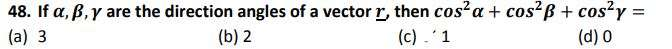3 / 61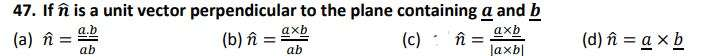4 / 61

If any two vectors of scalar triple product are equal, then its value is equal to

5 / 61

The angle in semi-circle is equal to:

6 / 617 / 61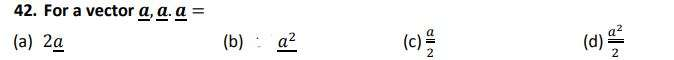8 / 619 / 61

. If the scalar product of three vectors is zero, then vectors are

10 / 6111 / 61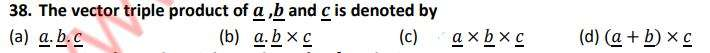12 / 61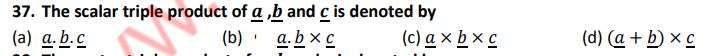13 / 6114 / 61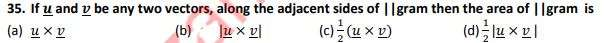15 / 6116 / 61

Cross product or vector product is defined

17 / 6118 / 6119 / 61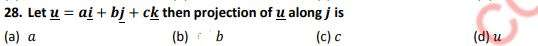20 / 6121 / 6122 / 61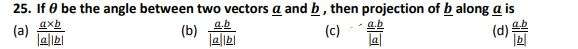23 / 6124 / 61

If the cross product of two vectors is zero, then the vectors must be

25 / 61

. If the dot product of two vectors is zero, then the vectors must be

26 / 61

Which of the following can be the direction angles of some vector

27 / 61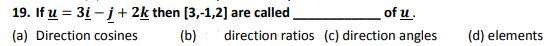28 / 6129 / 61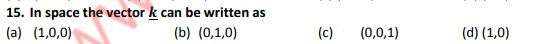30 / 6131 / 6132 / 61

A point P in space has __________ coordinates.

33 / 61

The lines joining the mid-points of any two sides of a triangle is always _____to the third side.

34 / 61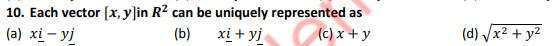35 / 61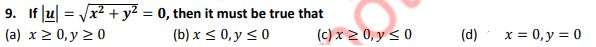36 / 61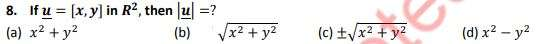37 / 61

The vector whose initial point is at the origin and terminal point is ? , is called

38 / 61

Parallelogram law of vector addition to describe the combined action of two forces, was used
by

39 / 61

Two vectors are said to be negative of each other if they have the same magnitude and
__________direction.

40 / 61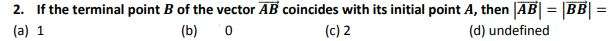41 / 61

The vector whose magnitude is 1 is called

42 / 61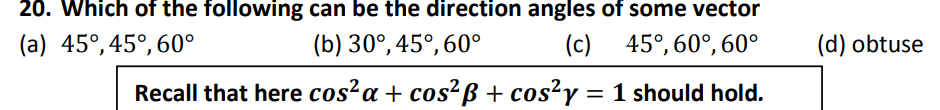43 / 6144 / 61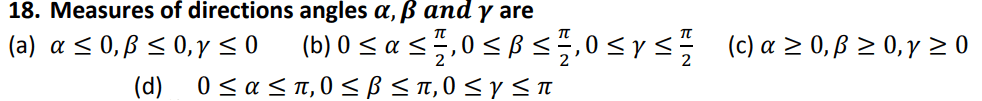45 / 61

The angles 𝜶, 𝜷, 𝒂𝒏𝒅 𝜸 which a non-zero vector 𝒓 makes with 𝒙 − 𝒂𝒙𝒊𝒔, 𝒚 − 𝒂𝒙𝒊𝒔 and
𝒛 − 𝒂𝒙𝒊𝒔 respectively are called_____________ of 𝒓.

46 / 61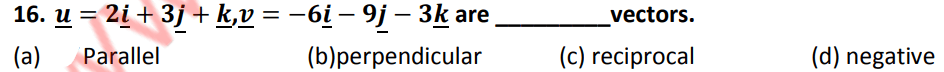47 / 61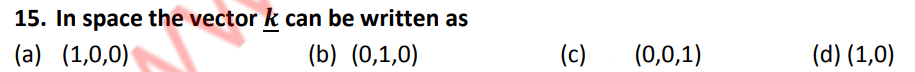48 / 6149 / 61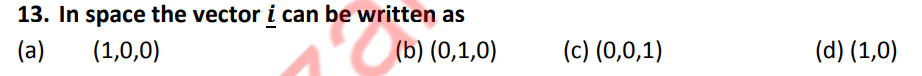50 / 61

A point P in space has __________ coordinates.

51 / 61

The lines joining the mid-points of any two sides of a triangle is always _____to the third side.

52 / 6153 / 61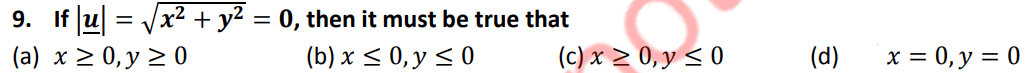54 / 61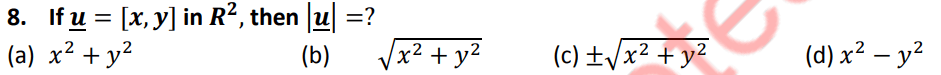55 / 6156 / 61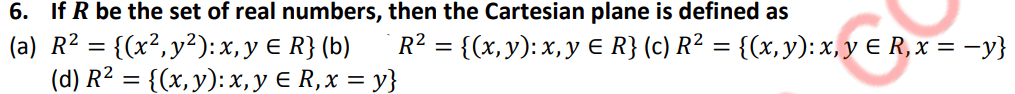57 / 61

The vector whose initial point is at the origin and terminal point is 𝑷 , is called

58 / 61

Parallelogram law of vector addition to describe the combined action of two forces, was used
by

59 / 61

Two vectors are said to be negative of each other if they have the same magnitude and
__________direction.

60 / 61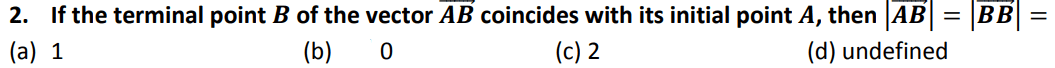61 / 61

The vector whose magnitude is 1 is called